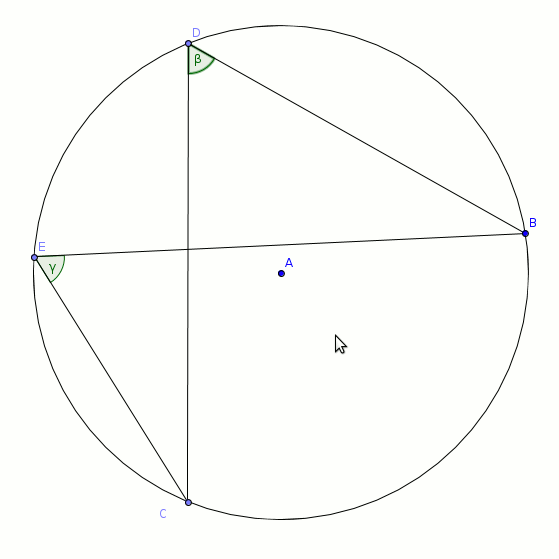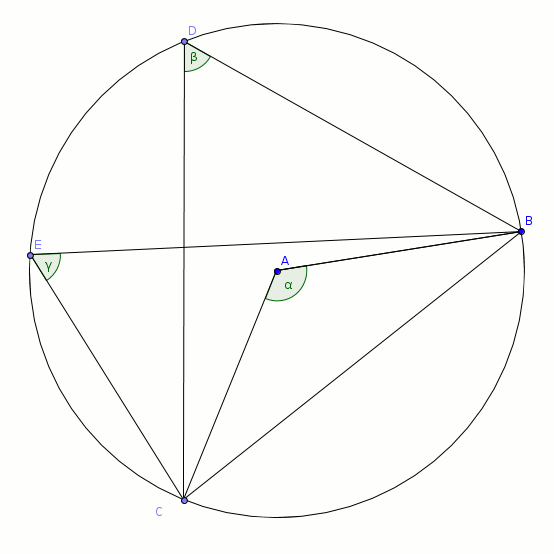# Third circle theorem proof

You are here: Home > Maths intro > Circle theorems first page > Angles in same segment proof

# 'Angles in the same segment are equal'Fig 1Fig 2

The 2 angles D and E are both sitting on the same piece of the circle, BC. ('Subtending the same minor arc BC' is a more mathsy way of putting it!).

In the second diagram, with the chord BC drawn, it is more obvious that angle D and E are in the same segment. It is also clear that A, the 'angle at the centre' BAC, is 'looking at' the same arc BC as the angles D and E.
So Theorem 1 applies: 'the angle at the centre is twice the angle at the circumference';

A=2D and A=2E, thus D=E.

ie, 'Angles in the same segment are equal'

QED